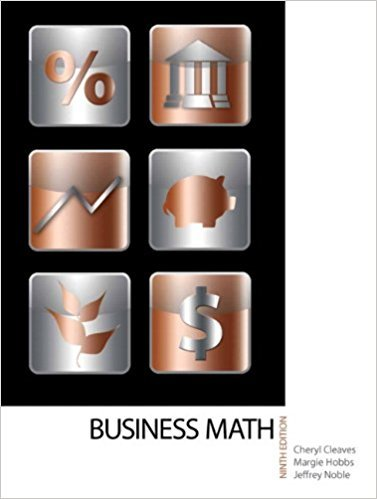×
×

# Solutions for Chapter 9-2: MARKUP BASED ON SELLING PRICE AND MARKUP COMPARISONS## Full solutions for Business Math, | 9th Edition

ISBN: 9780135108178Solutions for Chapter 9-2: MARKUP BASED ON SELLING PRICE AND MARKUP COMPARISONS

Solutions for Chapter 9-2
4 5 0 428 Reviews
27
4
##### ISBN: 9780135108178

This expansive textbook survival guide covers the following chapters and their solutions. This textbook survival guide was created for the textbook: Business Math, , edition: 9. Chapter 9-2: MARKUP BASED ON SELLING PRICE AND MARKUP COMPARISONS includes 22 full step-by-step solutions. Since 22 problems in chapter 9-2: MARKUP BASED ON SELLING PRICE AND MARKUP COMPARISONS have been answered, more than 18233 students have viewed full step-by-step solutions from this chapter. Business Math, was written by and is associated to the ISBN: 9780135108178.

Key Math Terms and definitions covered in this textbook
• Cayley-Hamilton Theorem.

peA) = det(A - AI) has peA) = zero matrix.

• Commuting matrices AB = BA.

If diagonalizable, they share n eigenvectors.

• Complex conjugate

z = a - ib for any complex number z = a + ib. Then zz = Iz12.

• Diagonalization

A = S-1 AS. A = eigenvalue matrix and S = eigenvector matrix of A. A must have n independent eigenvectors to make S invertible. All Ak = SA k S-I.

• Echelon matrix U.

The first nonzero entry (the pivot) in each row comes in a later column than the pivot in the previous row. All zero rows come last.

• Exponential eAt = I + At + (At)2 12! + ...

has derivative AeAt; eAt u(O) solves u' = Au.

• Gauss-Jordan method.

Invert A by row operations on [A I] to reach [I A-I].

• Hermitian matrix A H = AT = A.

Complex analog a j i = aU of a symmetric matrix.

• Incidence matrix of a directed graph.

The m by n edge-node incidence matrix has a row for each edge (node i to node j), with entries -1 and 1 in columns i and j .

• Independent vectors VI, .. " vk.

No combination cl VI + ... + qVk = zero vector unless all ci = O. If the v's are the columns of A, the only solution to Ax = 0 is x = o.

• Kronecker product (tensor product) A ® B.

Blocks aij B, eigenvalues Ap(A)Aq(B).

• lA-II = l/lAI and IATI = IAI.

The big formula for det(A) has a sum of n! terms, the cofactor formula uses determinants of size n - 1, volume of box = I det( A) I.

• Least squares solution X.

The vector x that minimizes the error lie 112 solves AT Ax = ATb. Then e = b - Ax is orthogonal to all columns of A.

• Left nullspace N (AT).

Nullspace of AT = "left nullspace" of A because y T A = OT.

• Minimal polynomial of A.

The lowest degree polynomial with meA) = zero matrix. This is peA) = det(A - AI) if no eigenvalues are repeated; always meA) divides peA).

• Normal matrix.

If N NT = NT N, then N has orthonormal (complex) eigenvectors.

• Outer product uv T

= column times row = rank one matrix.

• Pivot.

The diagonal entry (first nonzero) at the time when a row is used in elimination.

• Triangle inequality II u + v II < II u II + II v II.

For matrix norms II A + B II < II A II + II B II·

• Volume of box.

The rows (or the columns) of A generate a box with volume I det(A) I.

×